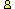CTK Exchange CTK Wiki Math Front Page Movie shortcuts Personal info Awards Terms of use Privacy Policy Cut The Knot! MSET99 Talk Games & Puzzles Arithmetic/Algebra Geometry Probability Eye Opener Analog Gadgets Inventor's Paradox Did you know?... Proofs Math as Language Things Impossible My Logo Math Poll Other Math sit's Guest book News sit's Recommend this siteCTK Exchange

 Subject: "Acute angled triangles" Previous Topic | Next Topic
 ConferencesThe CTK ExchangeCollege mathTopic #727Printer-friendly copyEmail this topic to a friend Reading Topic #727
johnm_12
Member since Mar-9-10
Mar-09-10, 11:14 AM (EST)"Acute angled triangles"

 If an acute angled triangle has sides of length x, y and z, where x<=y<=z, show that the corresponding angles, X, Y, and Z, can also form the sides of a triangle.

alexbCharter Member
2482 posts
Mar-09-10, 11:43 AM (EST)1. "RE: Acute angled triangles"
In response to message #0

 First of all, by the theorem of sines, x<=y<=z, implies X<=Y<=Z.With this in mind you only have to prove that Z <= X + Y. But this is obvious for an acute triangle; for then Z <= 90 <= X + Y.Note that for obtuse triangles the statement is not true.johnm_12guest
Mar-11-10, 06:21 PM (EST)

2. "RE: Acute angled triangles"
In response to message #1

 Nearly. You have to prove that Z 2Z (from above). Hence, X + Y > Z or Z < X + Y.Also, not true for Right-angled triangles as well as Obtuse.Interestingly (or not) I have never seen this in print before.I wonder if for all the years that mathematicians have been looking at triangles, they have just never noticed this.There are some interesting consequences here!!!E.g. 0 < π/2 - Z <= 3XY/2πAlso, if x, y, z is a non trivial solution to Fermat ...z^n = x^n + y^n, n>=2, then (x, y, z) forms an acute angled triangle only if n>=3.alexbCharter Member
2482 posts
Mar-11-10, 06:26 PM (EST)3. "RE: Acute angled triangles"
In response to message #2

 >Nearly. Yes, got it in a rush.>z^n = x^n + y^n, n>=2, then (x, y, z) forms an acute angled >triangle only if n>=3. This I have seen, although a reference escapes me.

 Conferences | Forums | Topics | Previous Topic | Next Topic
 Select another forum or conference Lobby The CTK Exchange (Conference)   |--Early math (Public)   |--Middle school (Public)   |--High school (Public)   |--College math (Public)   |--This and that (Public)   |--Guest book (Protected)   |--Thoughts and Suggestions (Public) Educational Press (Conference)   |--No Child Left Behind (Public)   |--Math Wars (Public)   |--Mathematics and general education (Public)You may be curious to have a look at the old CTK Exchange archive.